Thermodynamics and Propulsion
Subsections

# 14.3 Rocket Nozzles: Connection of Flow to Geometry

We have considered the overall performance of a rocket and seen that is directly dependent on the exit velocity of the propellant. Further, we have used the steady flow energy equation to determine the exhaust velocity using the combustion chamber conditions and the nozzle exit pressure. In this brief section, we will apply concepts from thermodynamics and fluids to relate geometrical (design) parameters for a rocket nozzle to the exhaust velocity.

We will make the following assumptions:

1. The propellant gas obeys the ideal gas law;
2. The specific heat is constant;
3. The flow in the nozzle is one-dimensional;
4. There are no losses due to friction;
5. There is no heat transfer;
6. The flow velocity in the combustion chamber is negligible (zero); and
7. The flow is steady.

## 14.3.1 Quasi-one-dimensional compressible flow in a variable area duct

Here we will use the ideal gas law,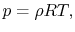and this expression for an isentropic flow,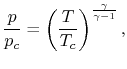where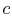will be used to refer to a chamber upstream of the duct. The first law for steady flow fromcan be written in terms of Mach number as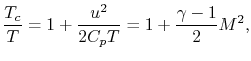where it has been assumed that the velocity atis small. From these latter two equations it follows that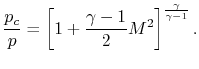Conservation of mass states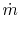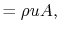and can be combined with the ideal gas law to produce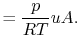All of these expressions can be combined to produceThe above equation relates the flow area, the mass flow, the Mach number and the stagnation conditions (conditions at). It is frequently rewritten in a non-dimensional form by dividing through by the value at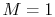(where the area atis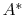):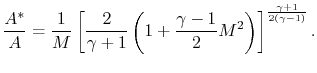This equation takes a form something like that shown in Figure 14.4.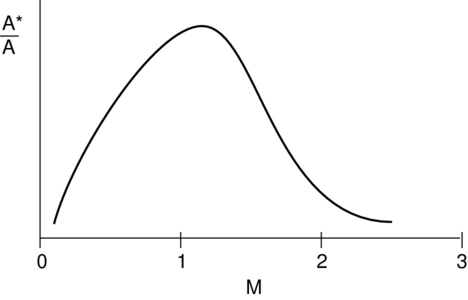## 14.3.2 Thrust in terms of nozzle geometry

We can use these equations to rewrite our expression for rocket thrust in terms of nozzle geometry (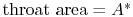), and exit area,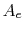. From before,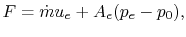where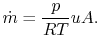Evaluating the mass flow at the throat, where,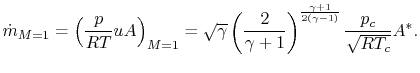The other terms in the thrust equation can be written in terms of chamber and exit conditions: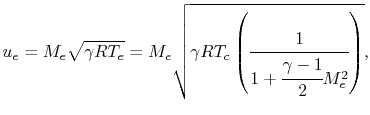and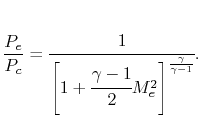We can now specify geometry (and) to determine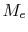, and then usewith the combustion chamber conditions to determine thrust and Isp.
UnifiedTP